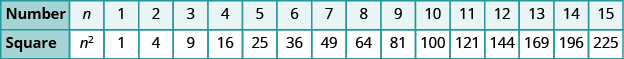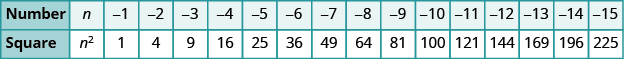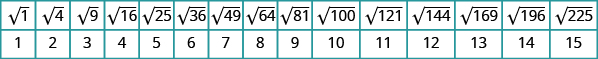## Modeling Squares and Finding the Square Root of a Number

### Learning Outcomes

• Simplify number statements involving perfect squares

## Simplify Expressions with Square Roots

To start this section, we need to review some important vocabulary and notation. Remember that when a number $n$ is multiplied by itself, we can write this as ${n}^{2}$, which we read aloud as “n squared”. For example, ${8}^{2}$ is read as “8 squared”.

We call $64$ the square of $8$ because ${8}^{2}=64$. Similarly, $121$ is the square of $11$, because ${11}^{2}=121$.

### Square of a Number

If ${n}^{2}=m$, then $m$ is the square of $n$.

## Modeling Squares

Do you know why we use the word square? If we construct a square with three tiles on each side, the total number of tiles would be nine.This is why we say that the square of three is nine.

${3}^{2}=9$

The number $9$ is called a perfect square because it is the square of a whole number.

The chart shows the squares of the counting numbers $1$ through $15$. You can refer to it to help you identify the perfect squares.### Perfect Squares

A perfect square is the square of a whole number.

What happens when you square a negative number?

$\begin{array}{cc}\hfill {\left(-8\right)}^{2}& =\left(-8\right)\left(-8\right)\\ & =64\hfill \end{array}$
When we multiply two negative numbers, the product is always positive. So, the square of a negative number is always positive.

The chart shows the squares of the negative integers from $-1$ to $-15$.Did you notice that these squares are the same as the squares of the positive numbers?

## Square Roots

Sometimes we will need to look at the relationship between numbers and their squares in reverse. Because ${10}^{2}=100$, we say $100$ is the square of $10$. We can also say that $10$ is a square root of $100$.

### Square Root of a Number

A number whose square is $m$ is called a square root of $m$.
If ${n}^{2}=m$, then $n$ is a square root of $m$.

Notice ${\left(-10\right)}^{2}=100$ also, so $-10$ is also a square root of $100$. Therefore, both $10$ and $-10$ are square roots of $100$.

So, every positive number has two square roots: one positive and one negative.

What if we only want the positive square root of a positive number? The radical sign, $\sqrt{\phantom{0}}$, stands for the positive square root. The positive square root is also called the principal square root.

### Square Root Notation

$\sqrt{m}$ is read as “the square root of $m\text{.''}$
If $m={n}^{2}$ then $\sqrt{m}=n$ for ${n}\ge 0$.We can also use the radical sign for the square root of zero. Because ${0}^{2}=0,\sqrt{0}=0$. Notice that zero has only one square root.
The chart shows the square roots of the first $15$ perfect square numbers.### example

Simplify: (a)  $\sqrt{25}$    (b)  $\sqrt{121}$

Solution
(a)  $\sqrt{25}=5$ since ${5}^{2}=25$

(b)  $\sqrt{121}=11$ since ${11}^{2}=121$

### try it

The following video shows several more examples of how to simplify the square root of a perfect square.

Every positive number has two square roots and the radical sign indicates the positive one. We write $\sqrt{100}=10$. If we want to find the negative square root of a number, we place a negative in front of the radical sign. For example, $-\sqrt{100}=-10$.

### example

Simplify. (a)  $-\sqrt{9}$   (b)  $-\sqrt{144}$

## Square Root of a Negative Number

Can we simplify $\sqrt{-25}?$ Is there a number whose square is $-25?$

${\left(\right)}^{2}=-25?$

None of the numbers that we have dealt with so far have a square that is $-25$. Why? Any positive number squared is positive, and any negative number squared is also positive. In the next chapter we will see that all the numbers we work with are called the real numbers. So we say there is no real number equal to $\sqrt{-25}$. If we are asked to find the square root of any negative number, we say that the solution is not a real number.

### example

Simplify: (a)  $\sqrt{-169}$   (b)  $-\sqrt{121}$.

## Contribute!

Did you have an idea for improving this content? We’d love your input.